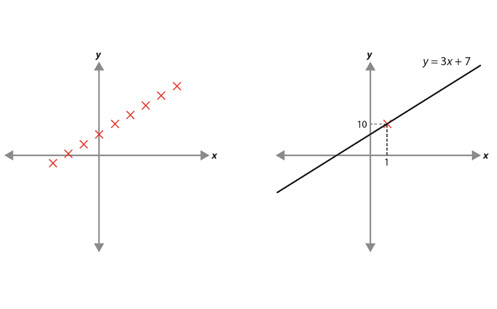# Solving linear equations

This paper explores the process of solving linear equations. It provides details of the knowledge students should have and some of the misconceptions they may hold. Examples given illustrate what these misconceptions may look like, how to address them, and support teachers in developing pedagogical and content knowledge.

Year level(s) Year 7, Year 8
Purpose Content knowledge
Keywords linear equation, solve

## Curriculum alignment

Strand and focus Algebra
Topics Linear relationships
AC: Mathematics (V9.0) content descriptions
AC9M7A03
Solve one-variable linear equations with natural number solutions; verify the solution by substitution

AC9M8A02
Graph linear relations on the Cartesian plane using digital tools where appropriate; solve linear equations and one-variable inequalities using graphical and algebraic techniques; verify solutions by substitution

Numeracy progression Number patterns and algebraic thinking (P7)